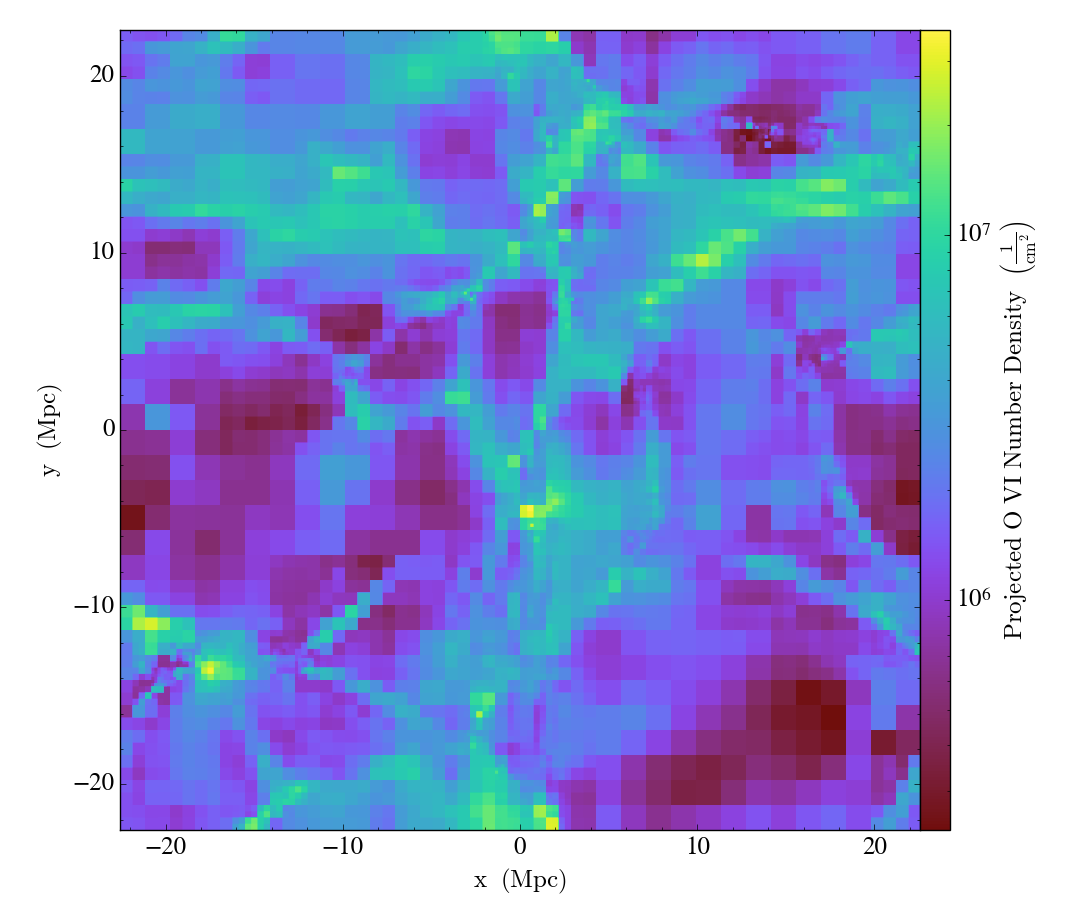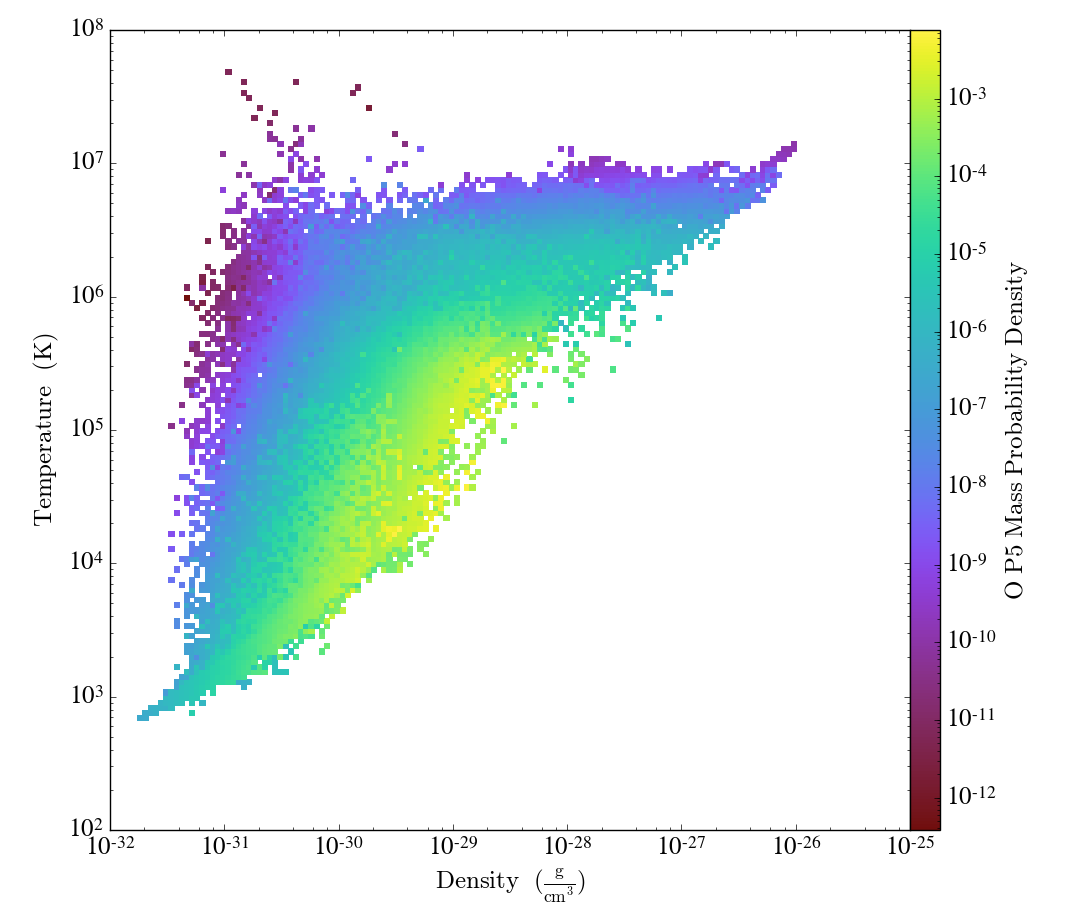In addition to being able to create absorption spectra, Trident can be used to postprocess datasets to add fields for ions not explicitly tracked in the simulation. These can later be analyzed using the standard yt analysis packages. This page provides some examples as to how these fields can be generated and analyzed.

## How does it work?

When you installed Trident, you were forced to download an ion table, a data table consisting of dimensions in density, temperature, and redshift. This ion table was constructed by running many independent Cloudy instances to approximate the ionization states of all ionic species of the first 30 elements. The ionic species were calculated assuming collisional ionization equilibrium based on different density and temperature values and photoionization from a metagalactic ultraviolet background unique to each ion table. The currently preferred ion table uses the Haardt Madau 2012 model. You can change your default ionization model by changing your config file (see: Manually Installing your Ionization Table), or by specifying it directly in the `ionization_table` keywords of the following functions.

By following the process below, you will add different ion fields to your dataset based on the above assumptions using the dataset’s redshift, and the values of density, temperature, and metallicity found for each gas parcel in your dataset.

## Generating species fields

As always, we first need to import yt and Trident and then we load up a dataset:

```import yt
import trident
fn = 'enzo_cosmology_plus/RD0009/RD0009'
```

To add ion fields we use the `add_ion_fields` function. This will add fields for whatever ions we specify in the form of:

• Ion fraction field. e.g. `Mg_p1_ion_fraction`

• Number density field. e.g. `Mg_p1_number_density`

• Density field. e.g. `Mg_p1_density`

• Mass field. e.g. `Mg_p1_mass`

Note

Trident follows yt’s naming convention for atomic, molecular, and ionic species fields. In short, the ionic prefix consists of the element and the number of times ionized it is: e.g. H I = `H_p0`, Mg II = `Mg_p1`, O VI = `O_p5` (p is for plus).

Let’s add fields for O VI (five-times-ionized oxygen):

```trident.add_ion_fields(ds, ions=['O VI'])
```

To show how one can use this newly generated field, we’ll make a projection of the O VI number density field to show its column density map:

```proj = yt.ProjectionPlot(ds, "z", "O_p5_number_density")
proj.save()
```

which produces:We can similarly create a phase plot to show where the O VI mass lives as a function of density and temperature:

```# we need to create a data object from the dataset to make a phase plot